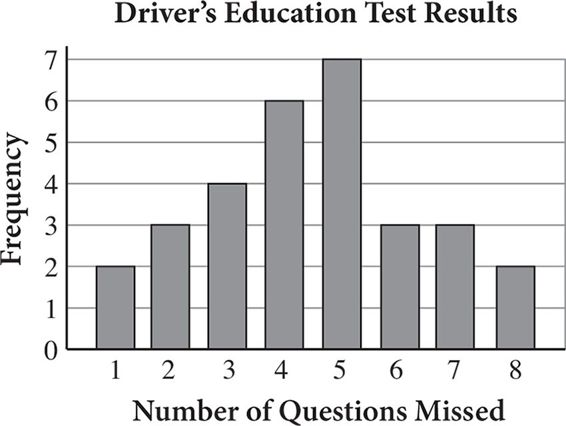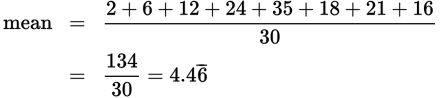# SAT Math Multiple Choice Question 565: Answer and Explanation

### Test Information

Question: 565

10.Mr. Juno took his driver's education class to the Department of Motor Vehicles to take their driver's license test. The number of questions missed by each student in the class is recorded in the bar graph above. Which of the following statements is true?

• A. More than half of the students missed 5 or more questions.
• B. The mean number of questions missed was between 4 and 5.
• C. More students missed 3 questions than any other number of questions.
• D. Thirty-six students from Mr. Juno's class took the driver's license test that day.

Explanation:

B

Difficulty: Medium

Category: Problem Solving and Data Analysis / Statistics and Probability

Strategic Advice: Always read the axis labels carefully when a question involves a chart or graph. Frequency, which is plotted along the vertical axis, tells you how many students missed the number of questions indicated under each bar.

Getting to the Answer: Evaluate each statement as quickly as you can.

Choice A: Add the bar heights (frequencies) that represent students that missed 5 or more questions: 7 + 3 + 3 + 2 = 15. Then, find the total number of students represented, which is the number that missed less than 5 questions plus the 15 you just found: 2 + 3 + 4 + 6 = 15, plus the 15 you already found, for a total of 30 students. The statement is not true because 15 is exactly half (not more than half) of 30.

Choice (B): This calculation will take a bit of time so skip it for now.

Choice C: The tallest bar tells you which number of questions was missed most often, which was 5 questions, not 3 questions, so this statement is not true.

Choice D: The number of students from Mr. Juno's class who took the test that day is the sum of the heights of the bars, which you already know is 30, not 36.

This means (B) must be correct. Mark it and move on to the next question. (Find the mean by multiplying each number of questions missed by the corresponding frequency, adding all the products, and dividing by the total number of students, which you already know is 30:The mean is indeed between 4 and 5.)